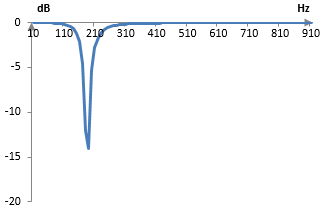# Notch filter

A notch filter is a frequency filter that passes all frequencies, except for a very narrow band of frequencies.

A notch filter is essentially a very narrow band stop filter. The opposite of a notch filter is a peak filter.

## An example of a notch filter and sound sample

See Orinj Notch filter and Orinj Notch filter 2 to hear sounds samples before and after a notch filter.

## Using notch filters

In music production, a notch filter can be used to remove a narrow band of overwhelming frequencies that make the sound offensive in some way – too boomy, nasal, and so on. Examples of such equalization are provided in the topic Equalizer.

A notch filter can also be used, for example, to remove the unwanted electrical equipment hum from a musical recording, should such hum appear in the recording. The frequency of an alternating electrical current is usually 50 Hz or 60 Hz and in this case a notch filter should be constructed to remove the frequencies of 50 Hz or 60 Hz from the signal respectively. An ideal notch filter would remove only those frequencies. Notch filters in practice would remove a narrow interval of frequencies around these frequencies.

## Example: a digital infinite impulse response notch filter

The function

$$H(S)=(\frac{S^2+1}{S^2+\frac{S}{Q}+1})^n$$

is the normalized transfer function of an infinite impulse response notch filter of order 2n, where 1/Q is the approximate width of the notch as described below.

Suppose that we want to compute the parameters of an infinite impulse response notch filter of order 2 and notch frequency of ωcc is the frequency that should be removed by the filter, expressed as an angular frequency between 0 and π). We make the substitutions S = s / ωc (where s = jω) and n = 1 and we use the bilinear transformation substitution s = 2 (z – 1) / (z + 1). The transfer function of the second order notch filter is

$$H(z)=\frac{(4+\omega_c^2)+(2\omega_c^2-8)z^{-1}+(4+\omega_c^2)z^{-2}}{(4+\frac{\omega_c}{Q}+\omega_c^2)+(2\omega_c^2-8)z^{-1}+(4-\frac{\omega_c}{Q}+\omega_c^2)z^{-2}}$$

Suppose that we want a notch at ωc = 0.6 with width 1/Q = 0.5 (technically, the notch will be at ωc = 2 arctan(0.6/2) ≈ 0.583, because of the warping of the frequency domain by the bilinear transformation). The transfer function of the second order notch filter will become

$$H(z)=\frac{0.935622-1562232\,z^{-1}+0.935622\,z^{-2}}{1-1562232\,z^{-1}+0.871245\,z^{-2}}$$

The filter itself is given by the formula

$$y(k) = 0.935622 x(k) – 1.562232 x(k-1) + 0.935622 x(k-2) + 1.562232 y(k-1) – 0.871245 y(k-2)$$

where x(k) and y(k) are the input and output signal at sample k respectively.

Suppose that the sampling frequency is 2000 Hz. The following is the magnitude response of the example notch filter of order 2.This filter passes all frequencies, except for a narrow band of frequencies (the "notch"). The frequency of the notch itself, given the sampling frequency of 2000 Hz, is ωc = (0.6 * 2000) / (2 π) = 191 Hz. The width of the notch is about 1/Q = (0.5 * 2000) / (2 π) = 159 Hz.

## Characteristics of notch filters

The notch filter above is just an example, and there are many ways to design digital or analog notch filters, which may have different characteristics. Notch filters are primarily characterized by the width and depth of the notch in their magnitude response. The width of the notch in the notch filter in the example above is approximately 160 Hz and its depth is approximately -14 dB. For this specific filter, a larger notch depth will also mean a larger notch width.

A notch filter may be designed to remove not only one specific notch frequency, but also harmonics of the notch frequency. For example, a notch filter at 60 Hz may be designed to also remove 120 Hz, 180 Hz, 240 Hz, and so on.

### Filtered HTML

• Freelinking helps you easily create HTML links. Links take the form of [[indicator:target|Title]]. By default (no indicator): Click to view a local node.